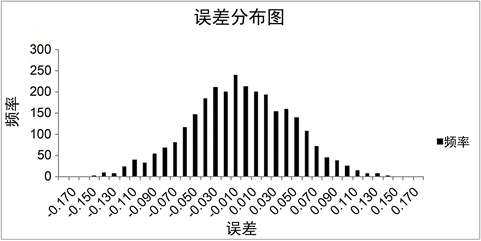#### 期刊菜单

Derivation and Validity Verification of Earthwork Measurement Error Formula
DOI: 10.12677/GST.2022.104020, PDF , HTML, XML, 下载: 164  浏览: 337  科研立项经费支持

Abstract: In practice, the methods of topographic mapping and earthwork calculation are relatively mature. There is a great deal of literature on surveying and mapping topographic maps by various methods. There is also a large number of literature on the calculation of earthwork. However, the research on how to map topographic maps to meet the accuracy of earthwork measurement is still insufficient. In this paper, from the perspective of grid elevation accuracy, the formulas of the relationship be-tween the number of grid elevation points, the accuracy error, and the relative error of earthwork measurement are derived, and the method of mathematical simulation was used to verify the effectiveness of the formula.

1. 引言

2. 土石方测量误差公式推导

${V}_{i}=S×\left({h}_{1}+{h}_{2}+\cdots +{h}_{n}\right)$ (1)

$V=n×h×S$ (2)

${m}_{v}^{2}={S}^{2}{m}_{{h}_{1}}^{2}+{S}^{2}{m}_{{h}_{2}}^{2}+\cdots +{S}^{2}{m}_{{h}_{n}}^{2}$ (3)

${m}_{v}=±\left(S×\sqrt{n}×{m}_{h}\right)$ (4)

${m}_{v}{}_{\mathrm{max}}=±3S×{m}_{h}$ (5)

${m}_{xd}=\frac{{m}_{v\mathrm{max}}}{v}\le ±\frac{3{m}_{h}}{\sqrt{n}×h}×100%$ (6)

3. 公式有效性验证

3.1. 模拟验证思路

1) 采用正态随机变量函数计算每一个高程点的误差返回值。验证误差返回值是否符合正态分布规律。

2) 误差返回值与原始高程数据值相加，生成新的模拟的测量高程点。采用模拟高程值计算土石方量，采用原始高程计算土石方量，求取二者差值。多次重复以上步骤，求取土石方量的最大误差，与采用公式计算的最大误差比较，验证公式的有效性。

3.2. 高程点模拟及其误差分布Figure 1. Error distribution

3.3. 公式验证

30次模拟的填挖土方量和填挖土方的相对误差数据见表1Table 1. The relative error of the filling and excavation quantity and the filling and excavation quantity in 30 simulations

$F=0.15/\sqrt{1092×0.704}=±0.645%$

$C=0.15/\sqrt{1699×0.580}=±0.627%$

4. 结论

  刘亮. 几种工程土石方量计算方法的比较[J]. 山西建筑, 2019, 45(8): 182-183.  张蒙, 王想红, 徐胜华, 肖冰, 文化立. 等高线约束的Delaunay三角网在土石方量计算中的应用[J]. 地理与地理信息科学, 2020, 36(4): 14-18.  中华人民共和国建设部及国家质量监督检验检疫总局. GB50026-2007工程测量规范[S]. 北京: 中国计划出版社, 2008.  中华人民共和国水利部. SL197-2013水利水电工程测量规范[S]. 北京: 中国水利水电出版社, 2013.  中华人民共和国住房和城乡建设部. CJJ/T8-2011城市测量规范[S]. 北京: 中国建筑工业出版社, 2011.  中华人民共和国水利部. SL52-2015水利水电工程施工测量规范[S]. 北京: 中国水利水电出版社, 2015: 69.